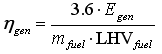# HOMER Pro 3.9

 Navigation: Glossary Generator Average Electrical EfficiencyType: Output Variable Units: % Symbol: ηgen

This is the average electrical efficiency of the generator over the year, defined as the electrical energy out divided by fuel energy in. HOMER uses the following equation to calculate the average electrical efficiency:where: Egen = the generator's total annual electrical production [kWh/yr] mfuel = the generator's total annual fuel consumption [kg/yr] LHVfuel = the lower heating value of the fuel [MJ/kg]

The factor of 3.6 in the above equation arises because 1 kWh = 3.6 MJ.